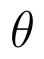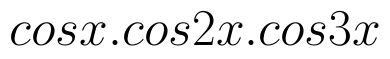Select Page

# CBSE MCQ Maths 12 Science Three Dimensional Geometry Solutions in English

CBSE MCQ Maths 12 Science Three Dimensional Geometry Solutions in English to enable students to get Solutions in a narrative video format for the specific question.

Expert Teacher provides CBSE MCQ Maths 12 Science Three Dimensional Geometry Solutions through Video Solutions in English language. This video solution will be useful for students to understand how to write an answer in exam in order to score more marks. This teacher uses a narrative style for a question from Three Dimensional Geometry not only to explain the proper method of answering question, but deriving right answer too.

Please find the question below and view the Solution in a narrative video format.

Question:

Solution Video in English:

## Similar Questions from CBSE, 12th Science, Maths, Three Dimensional Geometry

Question 1 : Find the equation of plane passing through the line of intersection of the planesandand passing through the point (3, -2, -1). Also, find the angle between the two given planes. (View Answer Video)

Question 2 : If the equation of a line AB isthen, find the direction cosines of a line parallel to AB. (View Answer Video)

Question 3 : If the linesandare perpendicular to each other, then find the value of P. (View Answer Video)

Question 4 : If a line marks anglesandwith x, y and z-axis respectively, whereis acute, then find. (View Answer Video)

Question 5 : Find the distance of the point (3, 4, 5) from the plane x + y + z = 2 measured parallel to the line 2x = y = z. (View Answer Video)

### Relations and Functions

Question 1 : If f is the greatest integer function and g is the modulus function . Write the value of g o f(-1/3) – f o g ( -1/3 ). (View Answer Video)

Question 2 : Number of binary operations on the set {a, b} are : (View Answer Video)

Question 3 : Ifis defined by, write f(f(x)). (View Answer Video)

Question 4 : The identity element for the binary operation * defined by a * b =, where a, b are the elements of a set of non-zero rational numbers, is, (View Answer Video)

Question 5 : Let * be the binary operation on N given by a * b = LCM of a and b. Find the identity of * in N. (View Answer Video)

### Continuity and Differentiability

Question 1 : If, findin terms of y alone. (View Answer Video)

Question 2 : Differentiate the function w.r.t.x. (View Answer Video)

Question 3 : Ifand, find. (View Answer Video)

Question 4 : Differentiate the function w.r.t.x. (View Answer Video)

Question 5 : Findfor the function. (View Answer Video)

### Integrals

Question 1 : Find the integral of the function. (View Answer Video)

Question 2 : Evaluate :(View Answer Video)

Question 3 : Evaluate :(View Answer Video)

Question 4 : Evaluate :. (View Answer Video)

Question 5 :. (View Answer Video)